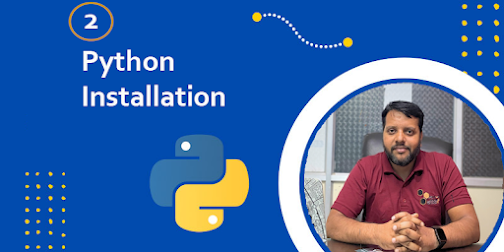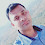49

#Python has an in-built IDLE that is best for python beginners.

1) PYTHON IDLE INSTALLATION Using Python .exe file
Python will be installed using two different ways
1) Offline using Python IDLE
2) Online using Jupyter
1)  Offline installation using IDLE:-
Step1st:-
for window 7 and before windows 7 o/s:-  Python 2.7 version should be installed.
After this version, we can install Python 3:
Step2nd:-
Open Python IDLE (Integrated Development Learning Environment)
By Default IDLE Provide Terminal mode which is for instant script execution for System administrator
step3rd:-
Click on File---> New File Option---> Write Python Script
print("Welcome in Python Script")
print("It is a script-based programming language which was created by GUIDO VAN RASSUM")
step4th:-
Save this file using the .py extension and click on the run option or press the F5 button.
Q) Write a program to calculate Simple Interest?
p=450000
r = 7.5
t =10
si = (p*r*t)/100
A = p+si
print("Total amount will be ",A)
print("Total interest will be ",si)
The solution of the SI program using the format():-
princ,rate,time = 25000,2,3  #multiple variable declration
si = (princ*rate*time)/100   #formula of SI
#print(si)                    #display output
#print("result of si is ",si)
print("result is princ={0}, rate={1} and time={2} and result={3}".format(princ,rate,time,si))

Write a program to perform the addition of two numbers?
a=100
b=200
c=a+b
print(c)
....................
........................................................................................................................................

2) Online using Jupyter

PYTHON INSTALLATION USING JUPYTER ONLINE TOOLS:-

Jupyter provides a Jupyter notebook that provides a completely online platform where we can easily execute Python Script Online.
We can execute python scripts on mobile applications also.
.......................................................................................................................................................
1)open browser and type jupyter.org/try
https://jupyter.org/try

2)
Click on the Try Jupyter Lab menu option
3) IT will redirect to Jupyter notebook with Jupyter Lab option
4)  Click on Python3 it will open the Jupyter notebook block
5)  Write code and execute this
WAP to SWAP Two numbers using Third Variable?
Solution 1:-
a=10
b=20
print(a,b)
temp=a
a=b
b=temp
print(a,b)
Solution 2:-
a=10
b=20
print("before swap a={0} and b={1}".format(a,b))
temp = a
a=b
b=temp
print("after swap a={0} and b={1}".format(a,b))
WAP to SWAP WITHOUT UISNG Third Variable?
a=10
b=20
a,b = b,a
print("a=",a," b= ",b)

Solution:-
a=123
print(a)
num1 = a%10  #3
a =(int)(a/10)   #12
num2 = a%10 #2
num3 = a//10 #1
res = num1*100+num2*10+num3*1
print(res)
WAP to display the middle number is a three-digit number?  a 145,154,451
WAP to calculate Square and Cube of any assigned number?

Tags

1.wap find square and cube of number
a=5
print(a*a)
print(a*a*a)

2.swap of two number using third variable in jupyter
a=10
b=20
print("before swap")
print(a,b)
temp=a
a=b
b=temp
print("after swap")
print(a,b)

3.a=100
b=200
a,b=b,a
print("a",a "b",b)

4.reverse number
a=123
print(a)
num1=a%10
a=(int)(a/10)
num2=a%10
num3=a//10
res=num1*100+num2*10+num*1
print(res)

5.Lokesh Rathore

Find the Middle number among three numbers
Solution :-
a = 145
b=a%10
a=a//10
b=a%10
print(b)

6.Lokesh Rathore

Square & Cube of any Number
Solution:-
a=5
b=a*a
c=a*a*a
print("The Square of 5 is ",b)
print("The Cube of 5 is ",c)

7.Lokesh Rathore

Reverse the Three Digit Number
Solution:-
a = 145
print("Number Beforte Reverse :- ",a)
b=a%10
a=a//10
c=a%10
d=a//10
e=b*100+c*10+d*1
print("Number After Reverse :- ",e)

8.#PYTHON (6 To 7 PM BATCH)
#To calculate Square and Cube of any assigned number.
z = int(input("Enter The No. :\t"))
z1 = int(input("For Square Press 2 or Press 3 for Cube :\t"))

if z1==2:

print(z**z1)
elif z1 ==3:

print(z**z1)

else:
print("Entered wrong Value")

9.Square and Cube of number
Square of the input number:-
x=int(input("Enter a Number:"))
y=(x*x)
print("The square of the number is:",y)

Cube of the input number:-
x=int(input("Enter a Number:"))
y=(x*x*x)
print("The cube of the number is:",y)

10.for i in range (1,6):
for j in range (i,6):
print(j,end='')

print()

11.a=10
b=20
a = a + b
b = a - b
a = a - b
print("a=",a," b= ",b)

12.a=5
s = a * a # Square = number * number
c = s * a # Cube = Square * number
print(" Square is ",s)
print("The Cube is ",c)

13.h,b=int(input("enter heigth of triangle:-")),int(input("enter base of triangle:-"))
area=(h*b)/2
print("area of triangle is:-",area)
l,w=int(input("enter length of rectangle:-")),int(input("enter width of ractangle:-"))
area1=l*w
print("area of ractangle is:-",area1)

14.num=int(input("enter any number:="))
print("reverse num is:-")
while num>0:
print(num%10)
num=num//10

15.#reverse 5 digits number without loop
mun=int(input("enter any number:-"))
a=mun%10
mun=mun//10
b=mun%10
mun=mun//10
c=mun%10
mun=mun//10
d=mun%10
mun=mun//10
e=mun%10
mun=mun//10
print(a,b,c,d,e)

16.#swap two numbers
a,b=int(input("enter the valu of a:-")),int(input("enter the valu of b:-"))
a,b=b,a
print("swapping values of a&b :-",a,b);

17.#Write a program to calculate Simple Interest?

p=20000
r=3
t=2

si=(p*r*t)/100
A=p+si

print('Total amount will be ',A)
print('Total amount will be ',si)

18.WAP to display the middle number is a three-digit number?

a=int(input("enter the value"))
b=int(input("enter the second value"))
c=a%10
a=a//10
c=a%10
d=b%10
b=b//10
d=b%10
print("the first mid no is",c ,"the second mid no is",d)

19.#Finding Simple Interest
p=2000
r=12
t=3
SI=(p*r*t)//100
print("the SI value is",SI)

20.a,b=34,12
print(a+b)

21.# program of swipeing 2 digit no.
a=20
b=34
ren=a
a=b
b=ren
print(a,b)

22.#program of swiping without third virable
a=input(" enter the first value")
b=input(" enter second value")
a,b = b,a
print(" the value of a is",a , "the value of b is ",b)

23.# Reverse three digit number.
num= 123
a=(num%10)#12.3
num1=(num//10)#12.3
b=(num1%10)#1.23
c=(num1//10)#1.23
rev= a*100+b*10+c*1
print( "the reverse no is ", rev)

24.# program to print middle number
a =int(input("Enter three digit number"))
b=a%10
a=a//10
b=a%10
print(b)

25.#WAP to calculate Square and Cube of any assigned number.
a=int(input("enter number for square and cube"))
square=(a*a)
print("the assgined square is",square)
cube=(a*a*a)
print("the Assgined cube is",cube)

26.#calculating Si unit
p=int(input("enter the value of p(principal)"))
r=float(input("enter the vlaue of r(rate)"))
t=int(input("enter the value of t(time)"))
si=(p*r*t)//100#simple intrest formula
print("the the value od si is",si)

27.a,b=34,12
print(a+b)

28.# program of swapeing 2 digit no.
a=int(input("enter the value of a"))
b=int(input("enter the value of b"))
c=a#c(remdom veriable to for storing value of a)
a=b
b=c
print(a,b)

29.#program of swiping without third virable
a=input(" enter the first value")
b=input(" enter second value")
a,b = b,a
print(" the value of a is",a , "the value of b is ",b)

30.# Reverse three degit no.
num=int(input("enter the value"))
a=(num%10)
num1=(num//10)
b=(num1%10)
c=(num1//10)
rev= a*100+b*10+c*1

print( "the reverse no is ", rev)

31.# program to print middle number
a =int(input("Enter three digit number"))
b=a%10
a=a//10
b=a%10
print(b)

32.#WAP to calculate Square and Cube of any assigned number.
a=int(input("enter number for square and cube"))
square=(a*a)
print("the assgined square is",square)
cube=(a*a*a)
print("the Assgined cube is",cube)

33.#calculate simple intrest
p=2000
r=9.8
t=8
si=float(input("enter simple interest"))
si=(p*r*t)%100
a=(p+si)
print(si)
print(a)

34.#swap teo no. using third variable.
a=20
b=30
print(a,b)
c=a
a=b
b=c
print(a,b)

35.#swap without using third variable.
a=10
b=30
a,b=b,a
print(a,b)

36.a=200
b=500
c=(a+b)
print(c)

37.# square or cube og given no
a=int(input("enter number:"))
b=a*a
print("square of given no is:",b)
c=a*b
print("cube of given no is:",c)

38.#shalu
a=12
b=10
area_tringal=0.5*a*b
print(area_tringal)
area_rectangle=a*b
print(area_rectangle)

39.#shalu
a=145
b=a%10
a=a//10
b=a%10
print(b)

40.#shalu
a=100
b=400
a,b=b,a
print(a,b)

#second method
a=10
b=20
temp=a
a=b
b=temp
print(a,b)

41.#shalu
h=6
s=h*h
c=h*h*h
print(s)
print(c)

42.#simple interest
p=3000
r=3.5
t=2
si=(p*r*t)/100
print("total simple intrest",si)
a=p+si
print("total amount",a)

43.a=2500
b=200
a+b

44.a=2500
b=200
a+b

45.#wap to swap without using third variable?
a=20
b=30
a=a+b
b=a-b
a=a-b
print(a,b)

46.# wap to reverse the three-digit number?
num=647
r1=num%10
num1=num//10
r2=num1%10
num2=num1//10
r3=num2%10
num3=num2//10
rev=r1*100+r2*10+r3*1
print(rev)

47.# wap to display the middle number is a three - digit number?
num=int(input("enter three digit number"))
r1=num%10
num1=num//10
r2=num1%10
print(r2)

48.#square and cube of the number?

#square of the input number
a=int(input("enter any number"))
b=a*a
print("square is",b)

# cube of the input number
x=int(input("enter any number"))
y=x*x*x
print("cube is",y)

49.a,b,c=10,20,30
a=a+b+c#60
c=a-(b+c)#(60-(20+30))=10
b=a-(b+c)#(60-(20+10))=30
a=a-(b+c)#(60-(30+10))=20
print("a={0} and b={1} and c={2}".format(a,b,c))